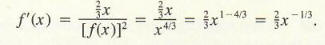Home | About | Contact | Disclaimer | Help

Algebra

Equations

Inequalities

Graphs

Numbers

Calculus

Matrices

Tutorials

Enter an expression and the variable or variables to differentiate with respect to and set the options. Then click the Differentiate button.

Expression
Variable(s)

[one per line]
Options

 Functions

[one per line]

Output Style
 Graphic image
 Text

# IMPLICIT DIFFERENTIATION

The equation y = x2 + 1 explicitly defines y as a function of x, and we show this by writing y = f (x) = x2 + 1. If we write the equation y = x2 + 1 in the form y - x2 - 1 = 0, then we say that y is implicitly a function of x. In this case we can find out what that function is explicitly simply by solving for y. Sometimes, however, we must deal with equations relating y to x that are so complicated that it is difficult or even impossible to solve for y in terms of x. (For example, try to solve for yin the equation yy + xy = 10.) If an equation implicitly defines y as a function of x, there is a way to find dy/dx without first explicitly finding y as a function of x, called implicit differentiation.

We will use the equation y - x2 - 1 = 0 to illustrate this technique. Instead of explicitly solving for y, assume that it would be possible to solve for y in terms of x; call the resulting function y(x) for simplicity. The equation can now be written as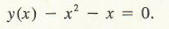Differentiating both sides with respect to x by means of the sum and power rules, we obtain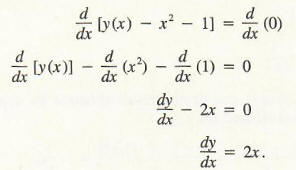This same result would be obtained by solving for y so that y = x2 + 1, from which dy/dx = 2x. In this example it is easier to first solve for y and then differentiate, but this will not always be the case.

Example 1

Find the slope of the tangent line to the graph of the equation xy - x = 1 at that point on the graph whose first coordinate is 1 (that is, corresponding to x = 1).

Solution

We must find dy/dx at x = 1. Assume y is a function of x, y = y(x). The relation now is xy(x) - x = 1.

Hence,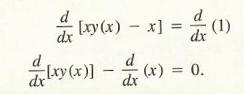and by the extended power rule,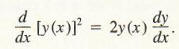Substituting these results into Formula (2), we obtain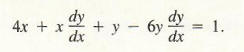We solve this equation for dy/dx: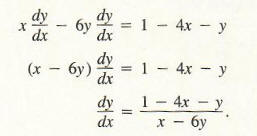Be careful! When applying Formula (3), keep in mind that the only values of x and y that can be substituted into the right-hand side of Formula (3) are those values that satisfy the original condition 2x2 + xy - 3y2 = x. For instance, we might substitute x = 1, y = 4 to obtain (dy/dx) = (1 - 4 - 2)/(1 - 12) = 5/1; however, (1, 2) is not a point on the graph of 2x2 + xy - 3y2 = x, so the calculation of dy/dx at this point is totally meaningless.

The equation in Example 2 can be written as - 3y2 + xy + (2x2 - x) = 0 and hence is a quadratic equation in y (an equation of the form Ay2 + By + C = o where A = -3, B = x and C = 2x2 - x). Hence, we could use the quadratic formula to solve this equation for y in terms of x, obtaining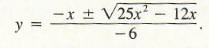Though we are able to find y explicitly in terms of x , the resulting expression is fairly complex, and it still might be best to find dy/dx implicitly as in Example 2.

Example 3 Find dy/dx if y4 + xy = 10.

Solving for y in terms of x is difficult if not impossible in this problem. However, by implicit differentiation, we obtain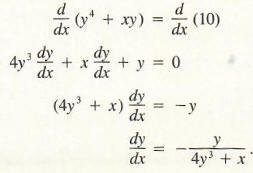The following example illustrates how implicit functions can be used to justify the fact that dxn /dx = nxn-1 i is valid when n is a rational number.

Example 4 Let f(x) = x2/3. Use implicit differentiation to show that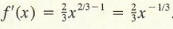Since f(x) = x2/3 , we obtain, by cubing,
[f(x)]3 = x2.

Differentiate with respect to x.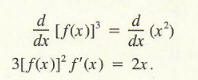Hence,